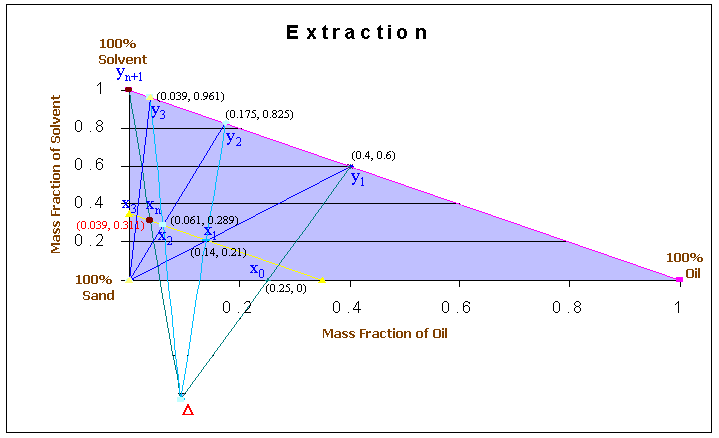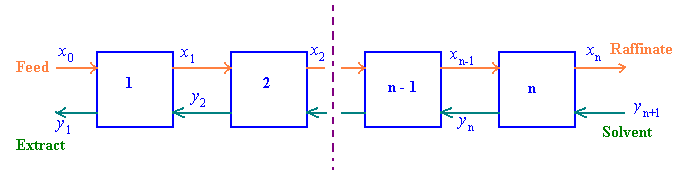### Solvent Extraction

60 tons per day of oil-sand (25 mass % oil and 75 mass % sand) is to be extracted with 40 tons per day of naphtha in a counter-current extraction battery. The final extract from the battery is to contain 40 mass % oil and 60 mass % naphtha and the underflow from each unit is expected to consists of 35 mass % solution and 65 mass % sand. If the overall efficiency of the battery is 50%, how many stages will be required?

Calculations: Basis: 1 day

Let us denote oil as A, sand as B and naphtha as S.

Since the oil-sand contains 25% of oil, xAo = 0.25 and xBo = 0.75

The final extract contains 40 percent of oil, and 60% of naphtha. Thus:

yA1 = 0.4 and yS1 = 0.6

The solvent used for extraction is pure and therefore, ySn+1 = 1.

For 60 tons of oil-sand, 75% is sand. This will come along with the final raffinate. i.e., Sand content is 60 x 0.75 = 45 tons. This amount contributes to 65% of the final raffinate. Therefore amount of raffinate = 45/0.65 = 69.23 tons.

The amount of solvent (naphtha) present in the final raffinate is obtained by overall mass balance for naphtha.

Overall Mass Balance:

feed + naphtha = raffinate + extract

i.e., 60 + 40 = 69.23 + extract

Therefore, extract = 60 + 40 -69.23 = 30.77 tons

Amount of naphtha entering = amount of naphtha in extract + amount of naphtha in raffinate

Therefore, 40 = 30.77 x 0.6 + 69.23 x fraction of naphtha in raffinate

i.e., Mass fraction of naphtha in raffinate = (40 - 30.77 x 0.6)/69.23 = 0.311

and mass fraction of oil in raffinate = 1 - 0.65 - 0.311 = 0.039

Thus xAn = 0.039, xBn = 0.65 and xSn = 0.311.

The mass fraction of sand in the underflow is constant and equal to 0.65(given). The composition of the underflow is represented, on the diagram, by a straight line parallel to the hypotenuse of the triangle with an intercept of (1 - 0.65) = 0.35 on the two main axis.

The difference point D is obtained as the point of intersection, by drawing two lines connecting x0 and y1 and xn and yn+1. For the stage 1, the underflow of composition x1 will contain insoluble solid mixed with solution of the same concentration as that in overflow y1, on the assumption that equilibrium conditions are reached.

The graphical construction to find the number of stages is shown in the following diagramFrom the diagram it can be seen that about 2.5 theoretical stages is required for this separation. The countercurrent extraction scheme is as given below:And the actual number of stages = theoretical number of stages/efficiency = 2.5/0.5 = 5

Ref: Chemical Engineering, Coulson & Richardson Vol-2, Butterworth-Heinemann